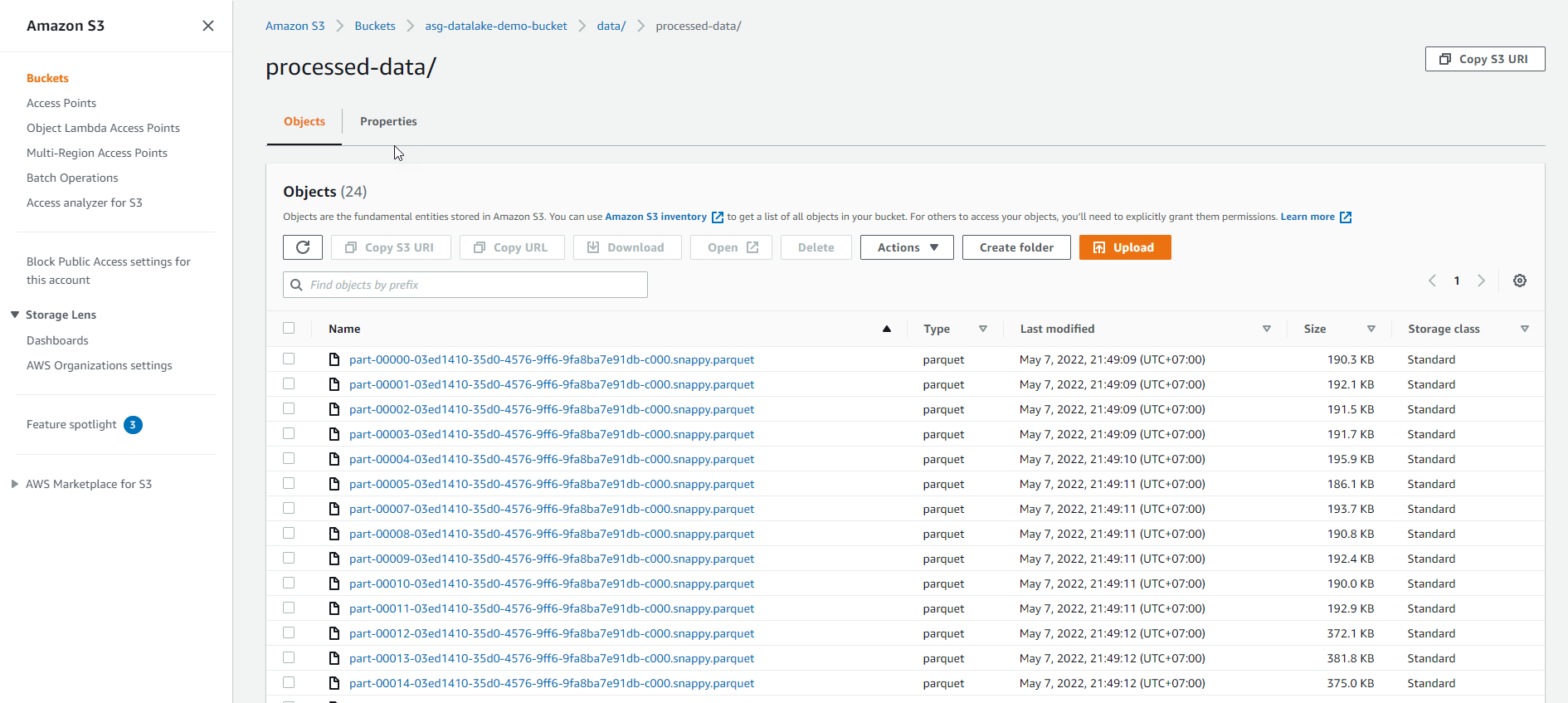# Create SageMaker Notebook

#### Create SageMaker Notebook

You can create SageMaker Notebook in 2 ways (choose 1 of 2):

• Find AWS Glue
• Select AWS Glue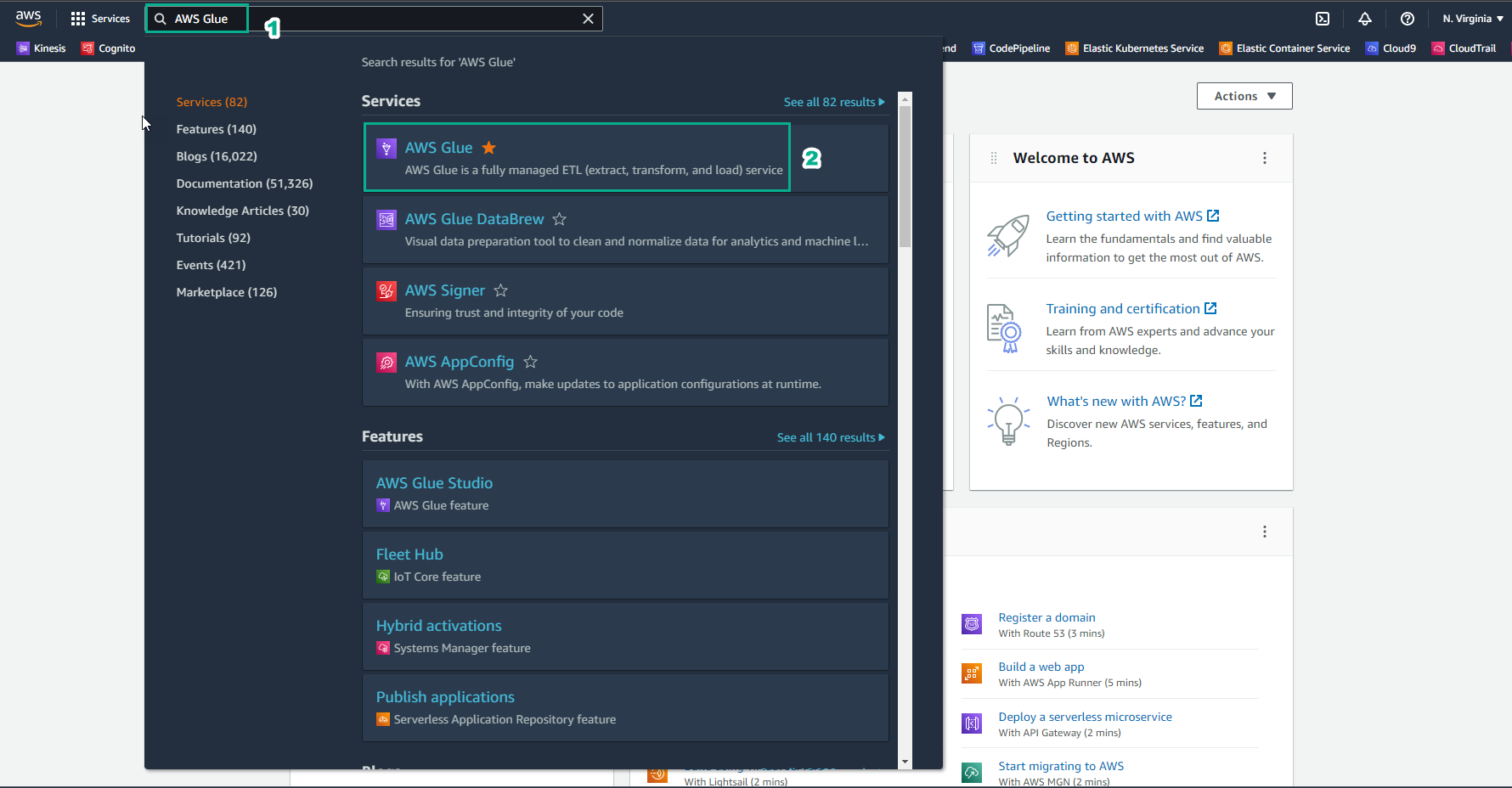1. Select Notebooks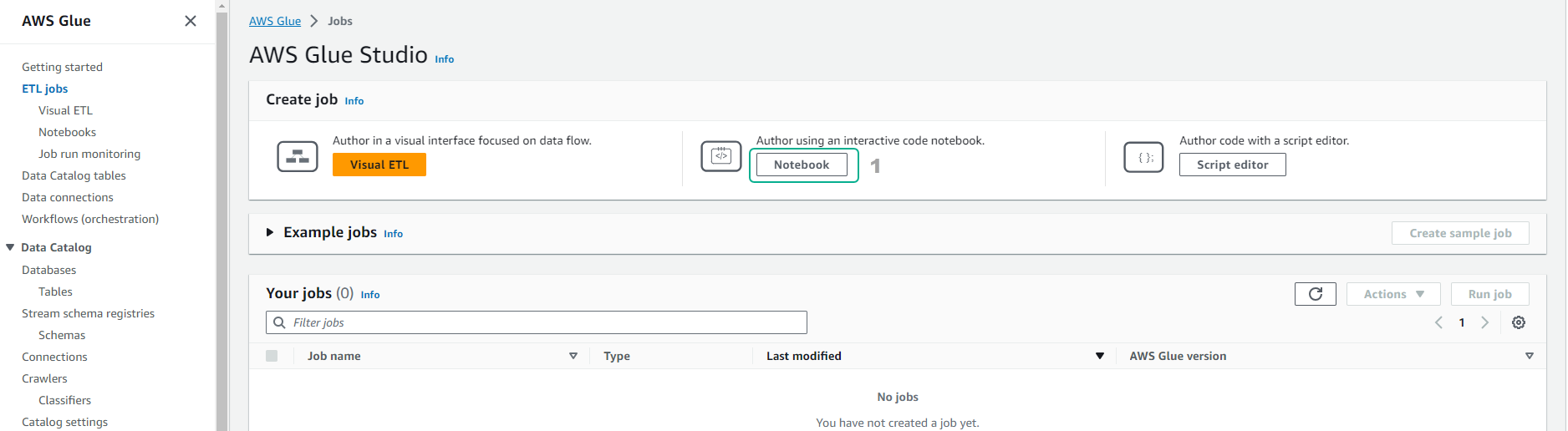1. Enter the notebook name as `notebook`
• Select IAM role
• Select Start notebook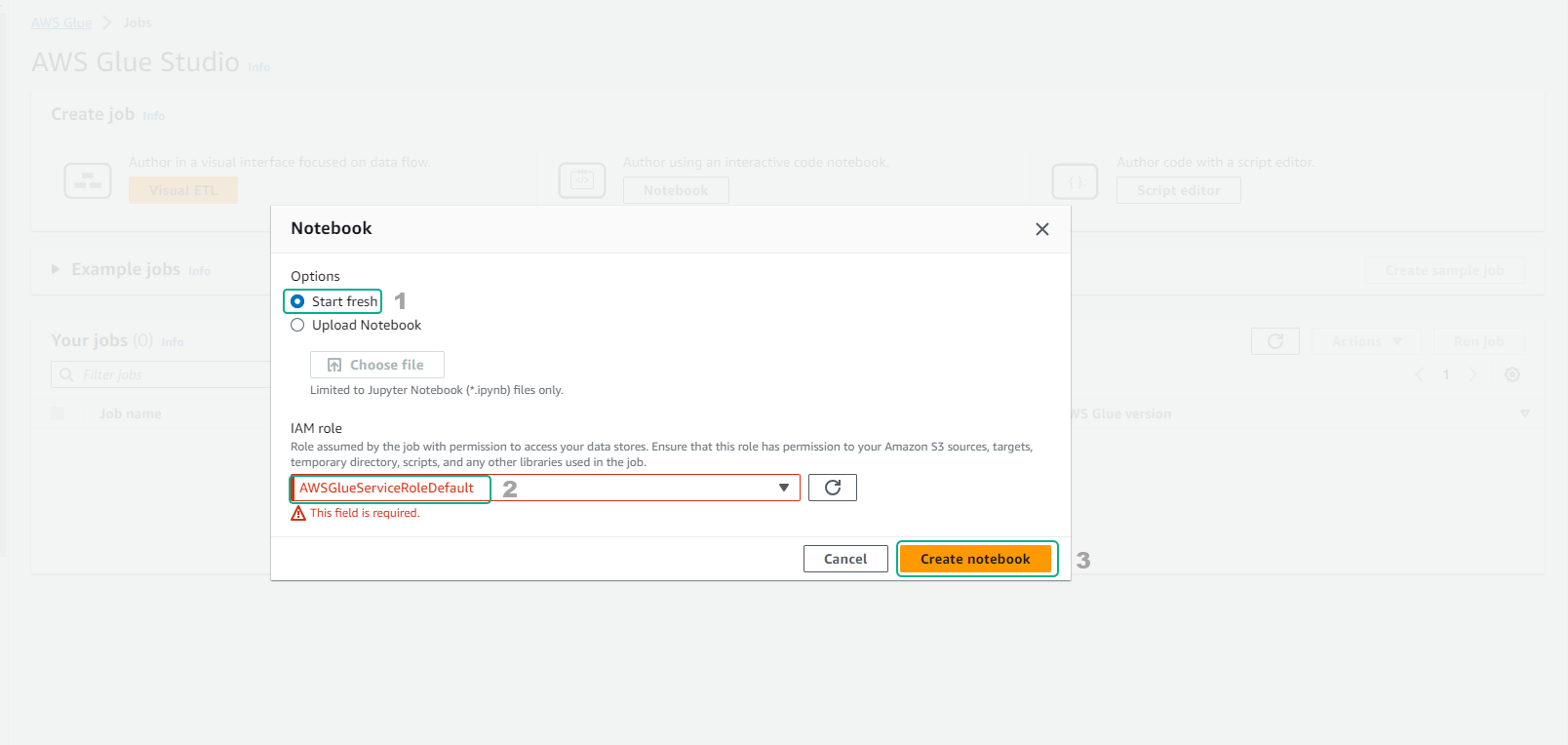1. Wait for about 2-3 minutes to complete the notebook.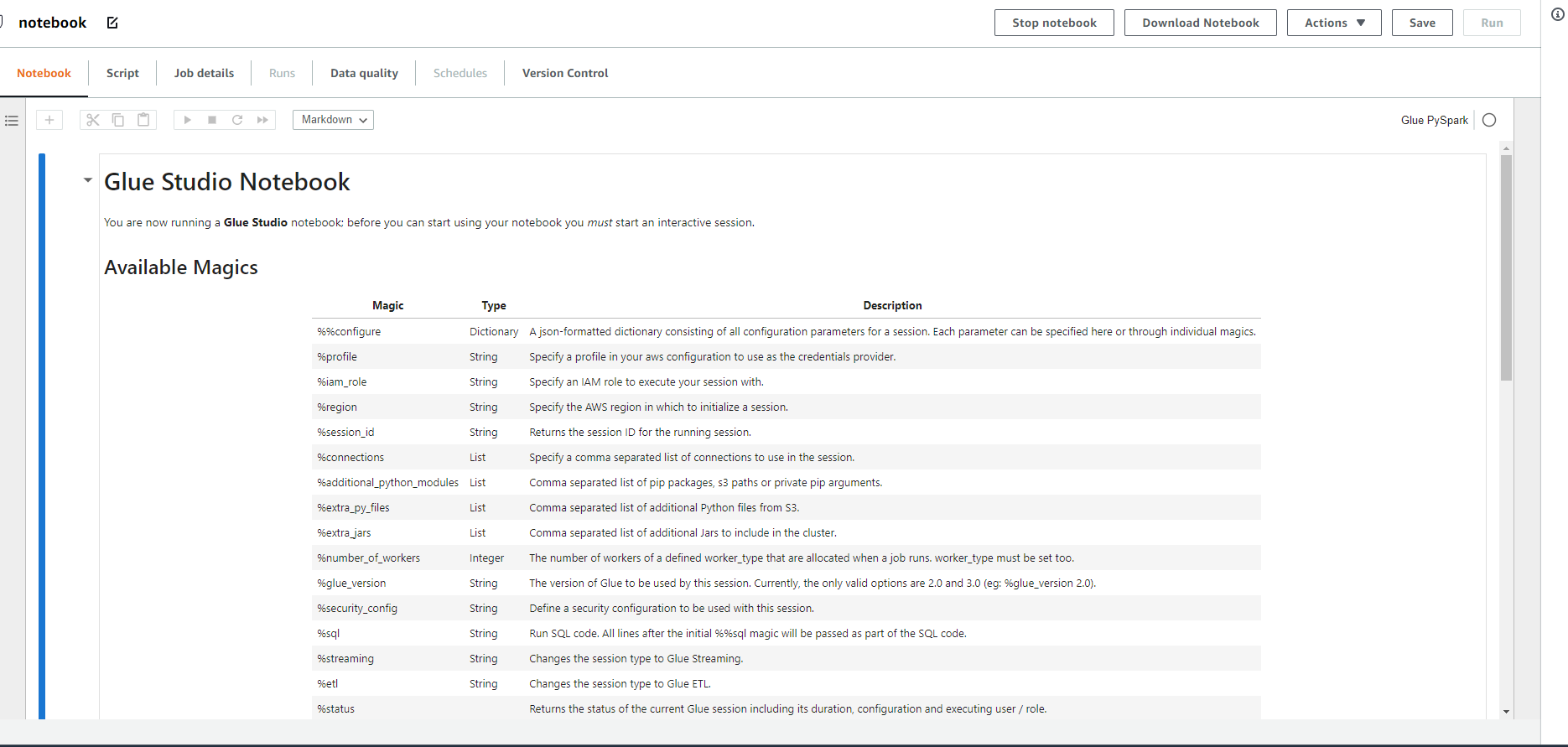1. You Run the first code to initialize Session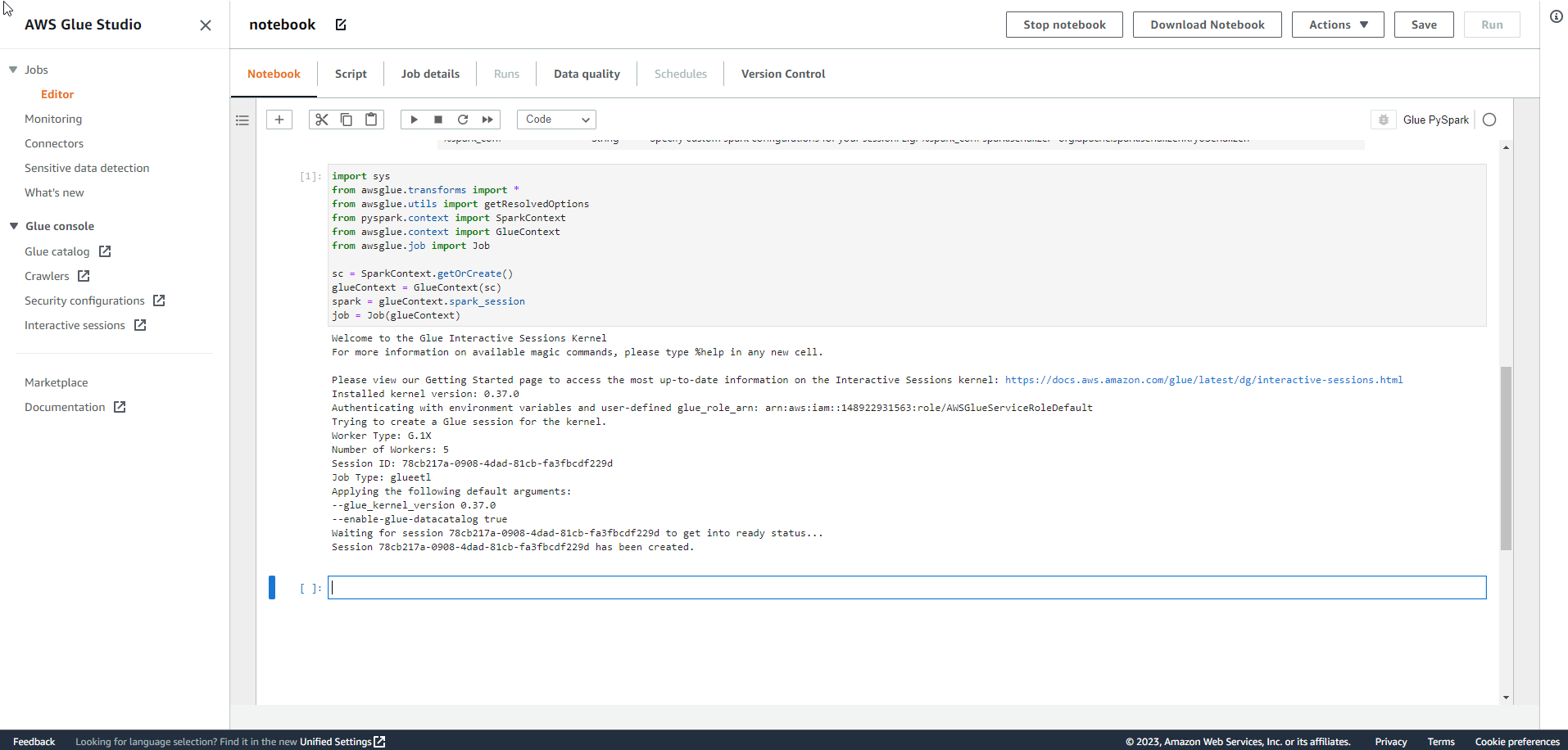• Use the keyboard shortcut Ctrl + S to Save the notebook file as .ipynb
• Then copy and run the code line by line from the notebook.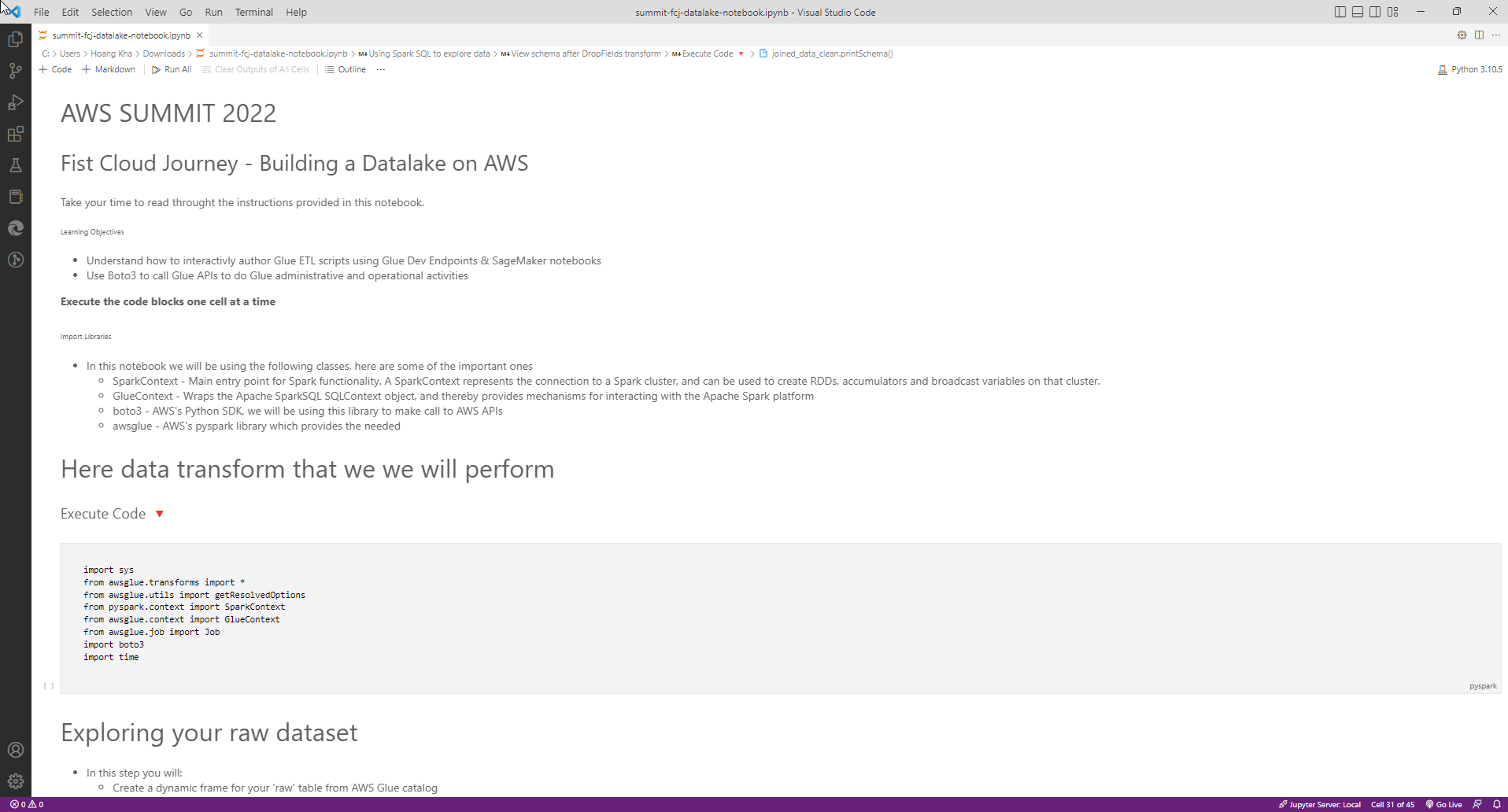1. So you have completed the initialization of 1 Interactive Session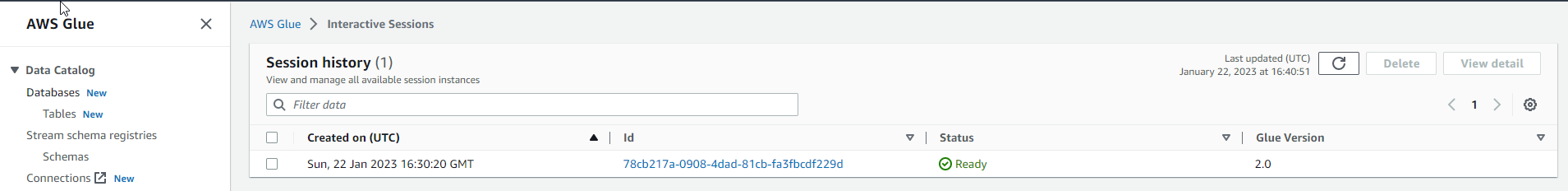#### Way 2 to create Notebook

• Select Jobs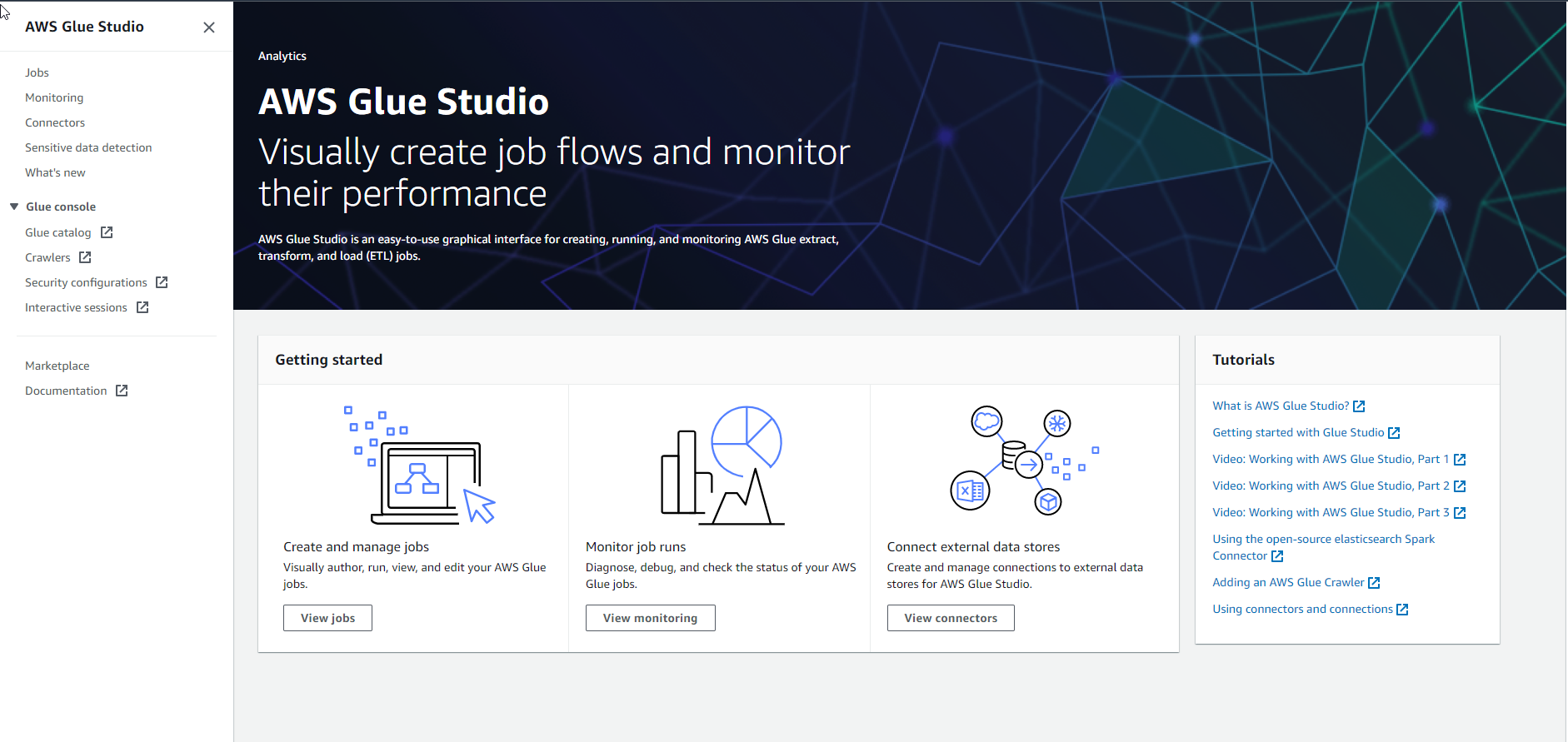1. In the Jobs interface
• Select Jupyter notebook
• Select Upload and edit an existing notebook
• Select Create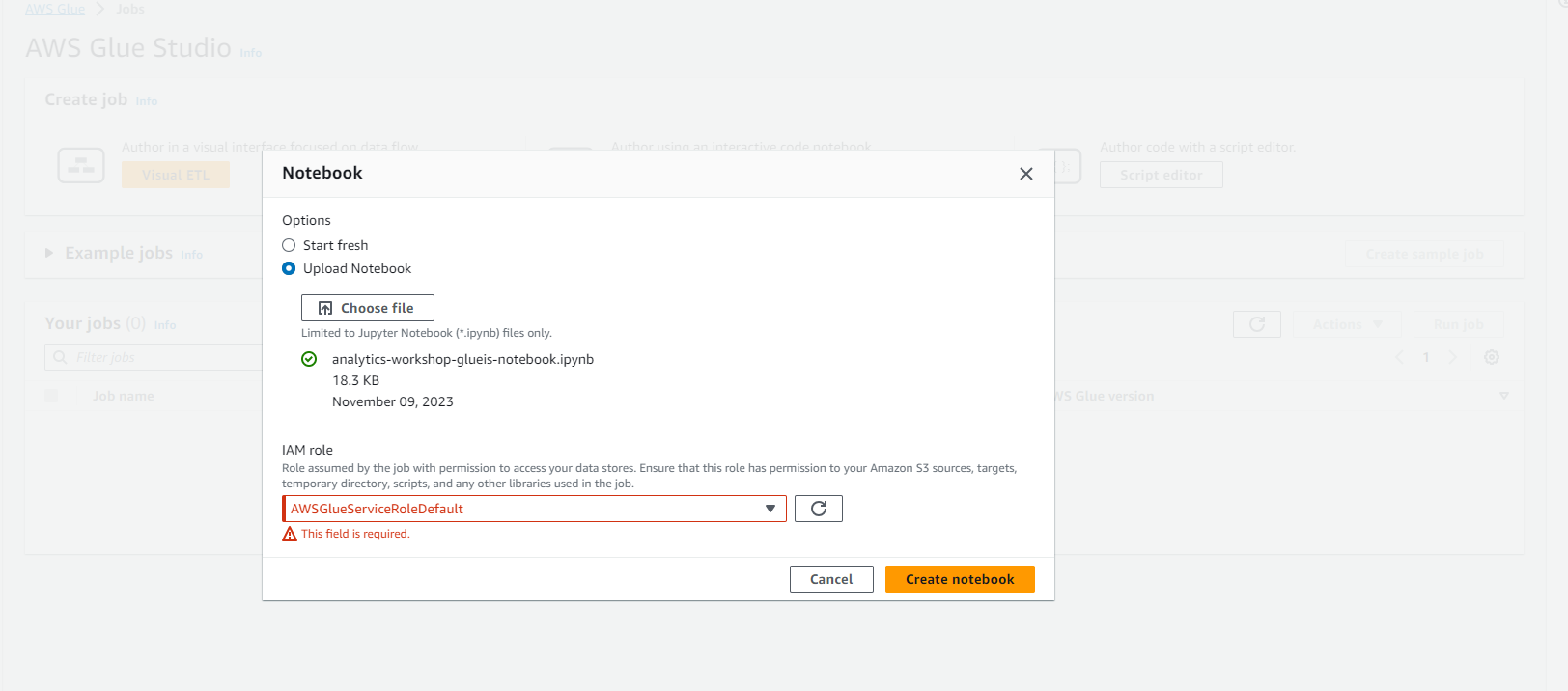1. Finish creating a Notebook.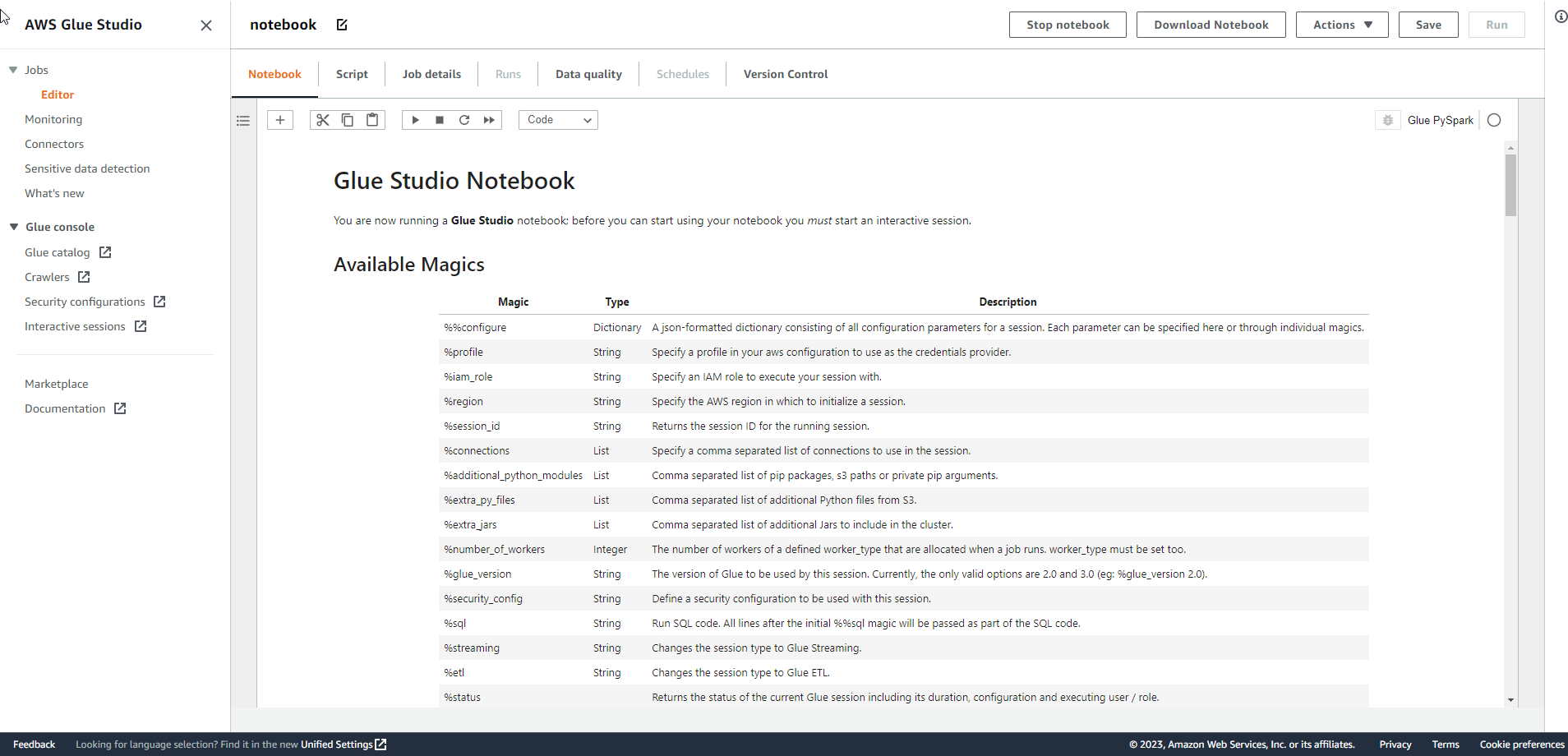1. Complete session initialization.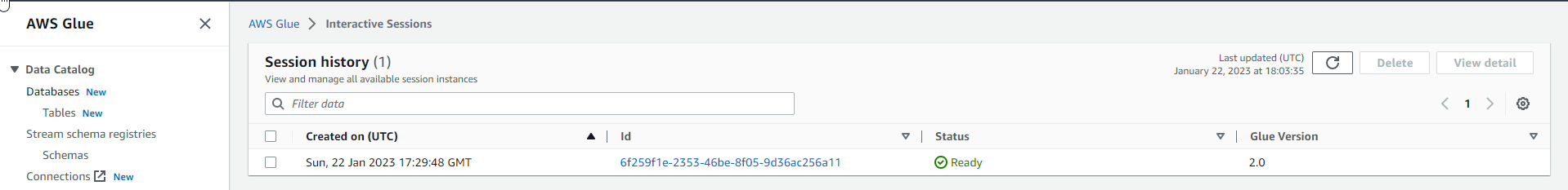#### Run and interpret the code.

1. In the Notebook interface
• First, we will import libraries

• SparkContext
• GlueContext
• boto3
• awsglue
``````import sys
from awsglue.transforms import *
from awsglue.utils import getResolvedOptions
from pyspark.context import SparkContext
from awsglue.context import GlueContext
from awsglue.job import Job
import boto3
import time
``````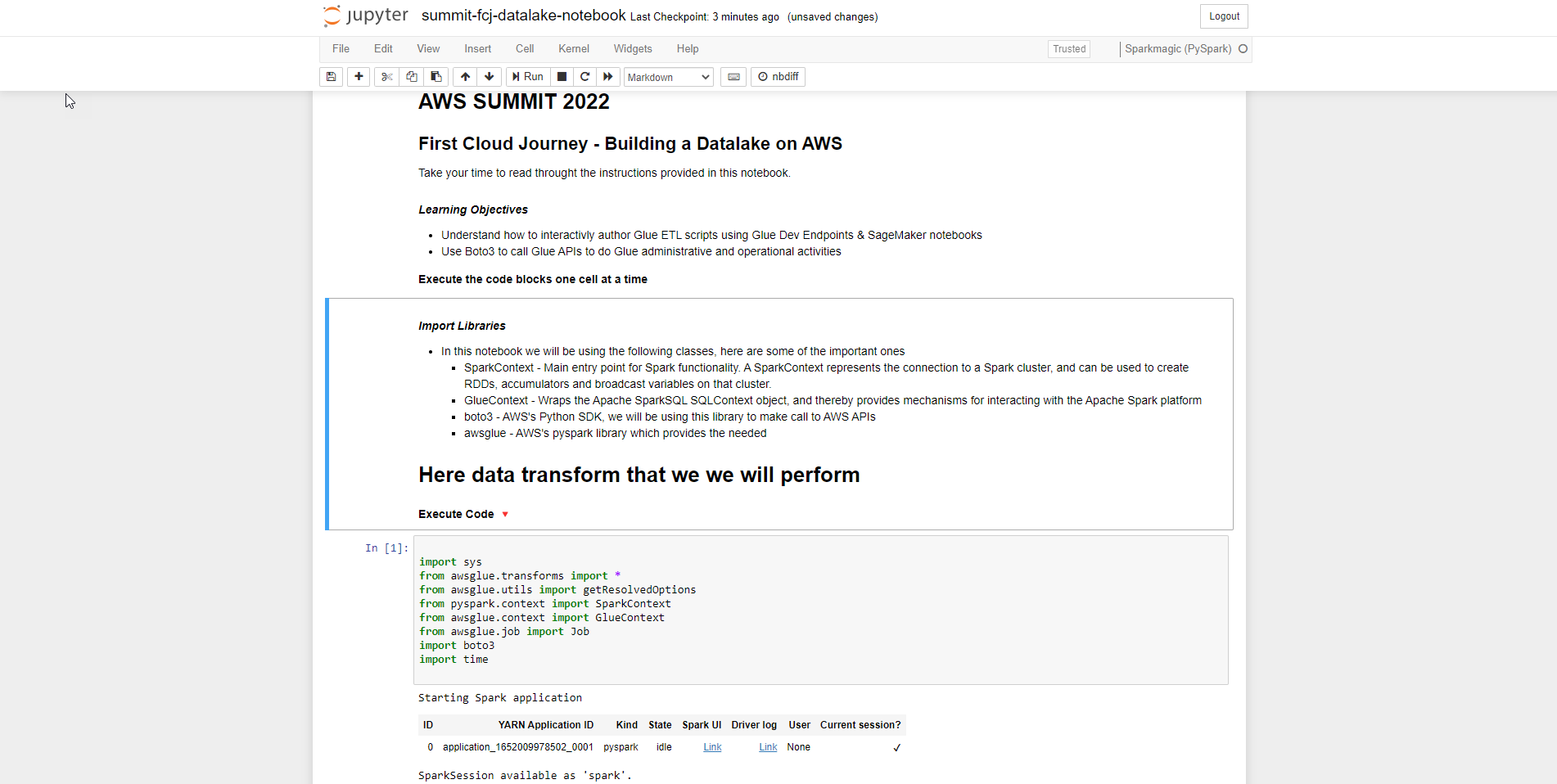1. Next we start to explore the data
• See an introduction to Glue Dynamics Frames Basics
``````glueContext = GlueContext(SparkContext.getOrCreate())
spark = glueContext.spark_session
``````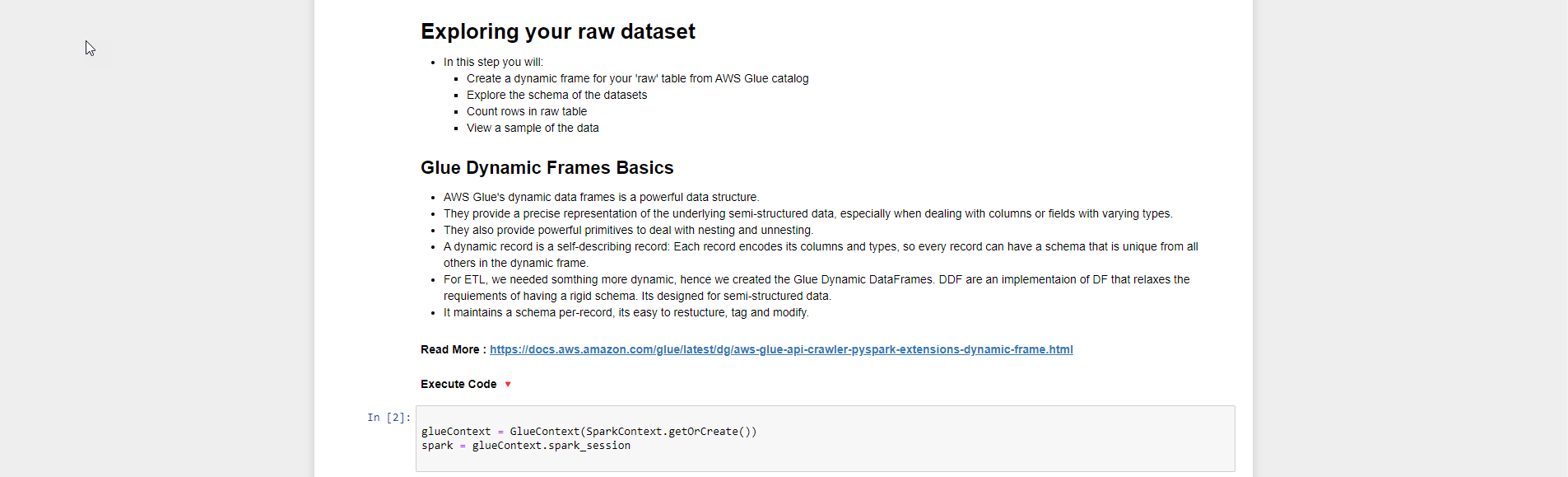1. Create dynamic frame for the raw2022 table (the table name can be changed by you so you can customize it at runtime) from the AWS Glue catalog
• You can refer to documents from Read More
``````raw_data = glueContext.create_dynamic_frame.from_catalog(database = "summitdb", table_name = "raw2022")

reference_data = glueContext.create_dynamic_frame.from_catalog(database = "summitdb", table_name = "reference_data")
``````1. Next step we look at the schema of dynamic frame
• Use the command printSchema
``````raw_data.printSchema()
``````
``````reference_data.printSchema()
``````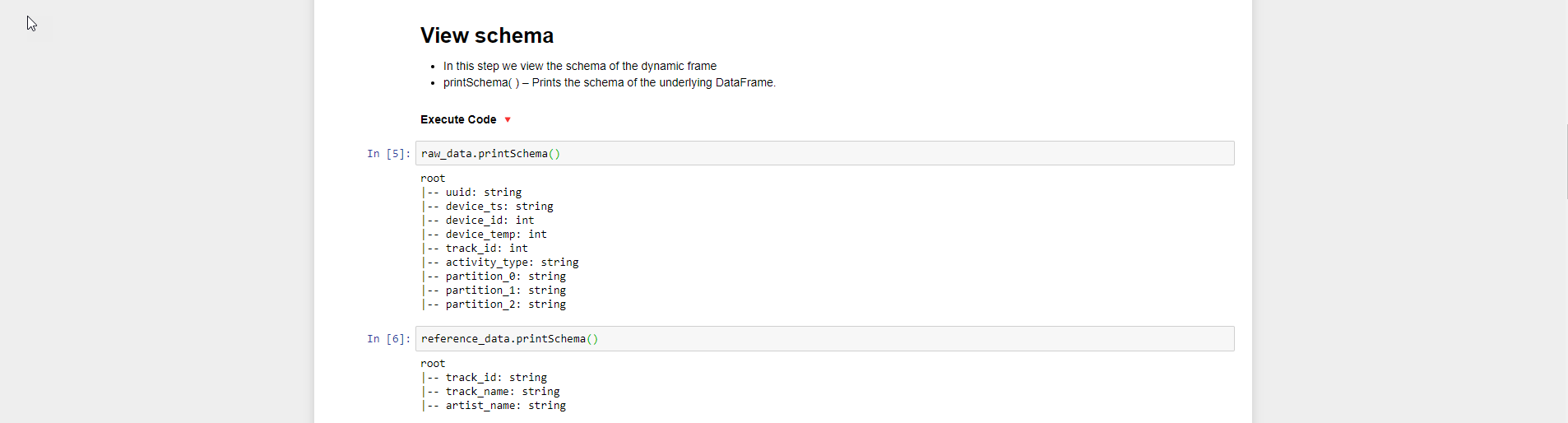1. Then we count the number of records in the dataframe using the count() function
``````print('raw_data (Count) = ' + str(raw_data.count()))
print('reference_data (Count) = ' + str(reference_data.count()))
``````1. To show sample records we use the show() function and pass in the number of records to show. In this lab we show 5 records from Dataframe
``````raw_data.toDF().show(5)
``````
``````reference_data.toDF().show(5)
``````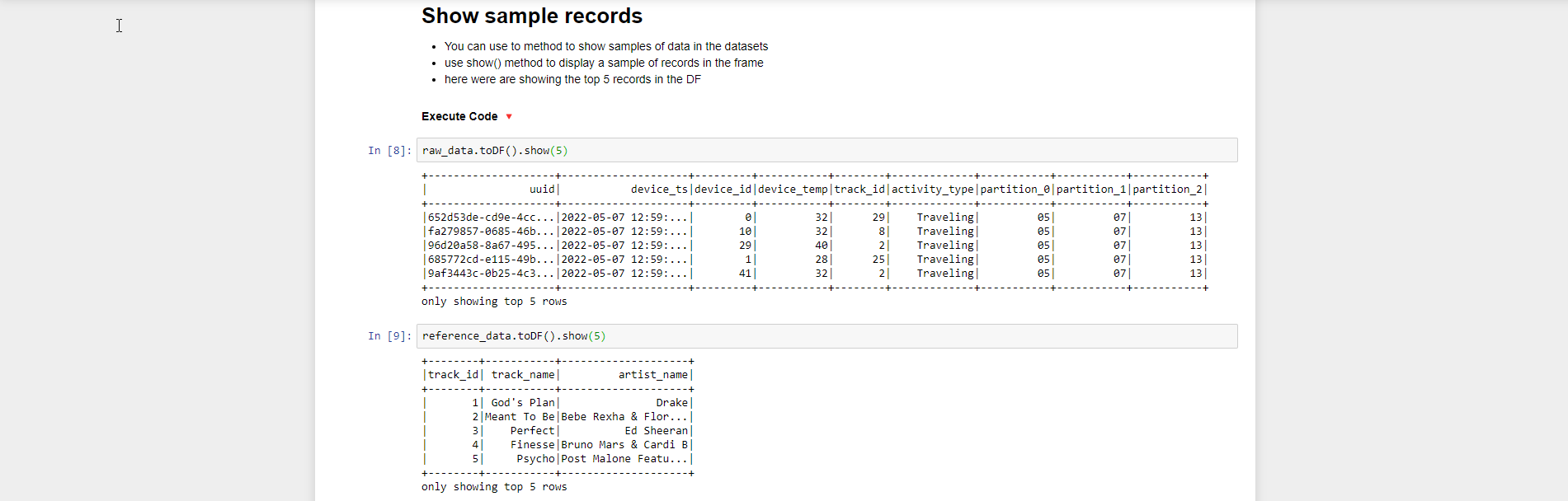1. Next, we will use Spark SQL to explore data
• Spark SQL - Filtering & Counting - activity_type = Running
``````# Adding raw_data as a temporary table in sql context for spark

raw_data.toDF().createOrReplaceTempView("temp_raw_data")

# Running the SQL statement which
runningDF = spark.sql("select * from temp_raw_data where activity_type = 'Running'")
print("Running (count) : " + str(runningDF.count()))

runningDF.show(5)
``````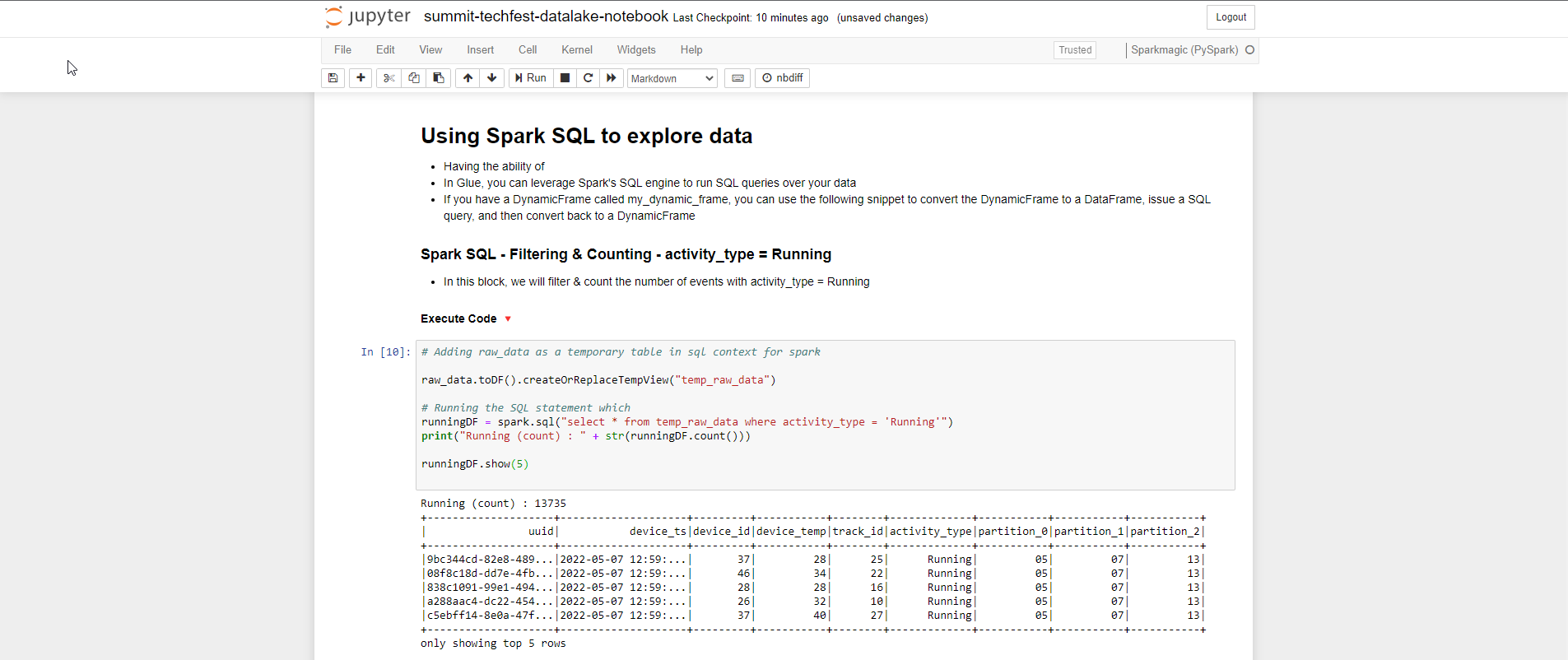1. Spark SQL - Filtering & Counting - activity_type = Working
``````# Running the SQL statement which
workingDF = spark.sql("select * from temp_raw_data where activity_type = 'Working'")
print("Working (count) : " + str(workingDF.count()))

workingDF.show(5)
``````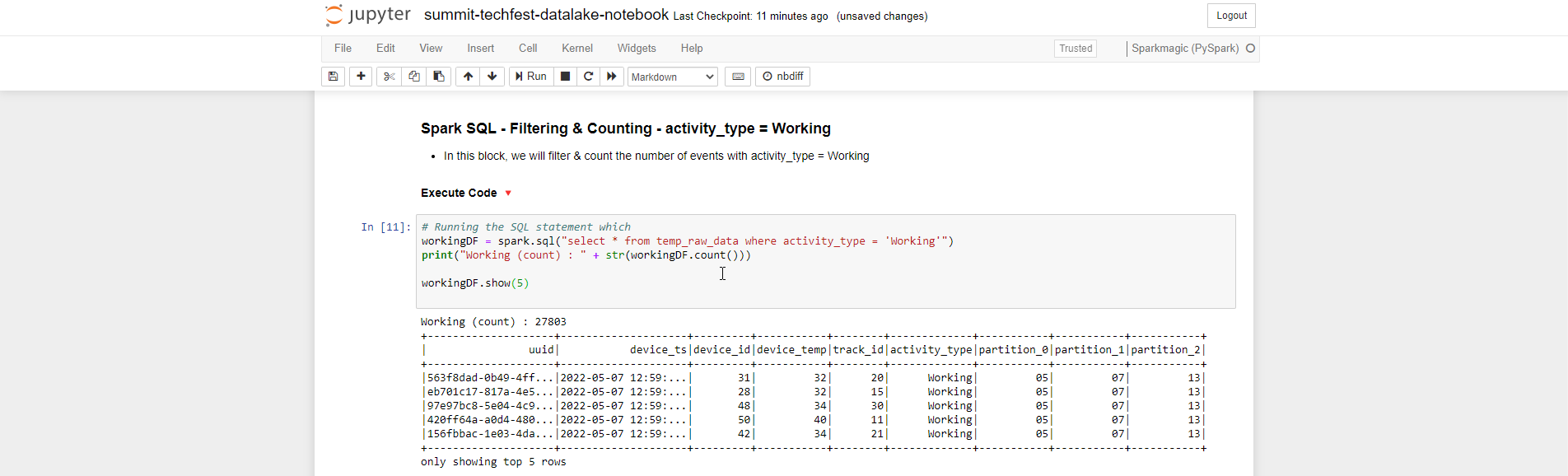1. Next step, we perform transform using Filter() function
• Glue Transforms - Filtering & Counting - activity_type = Running
``````def filter_function(dynamicRecord):
if dynamicRecord['activity_type'] == 'Running':
return True
else:
return False
runningDF = Filter.apply(frame = raw_data, f = filter_function)

print("Running (count) : " + str(runningDF.count()))
``````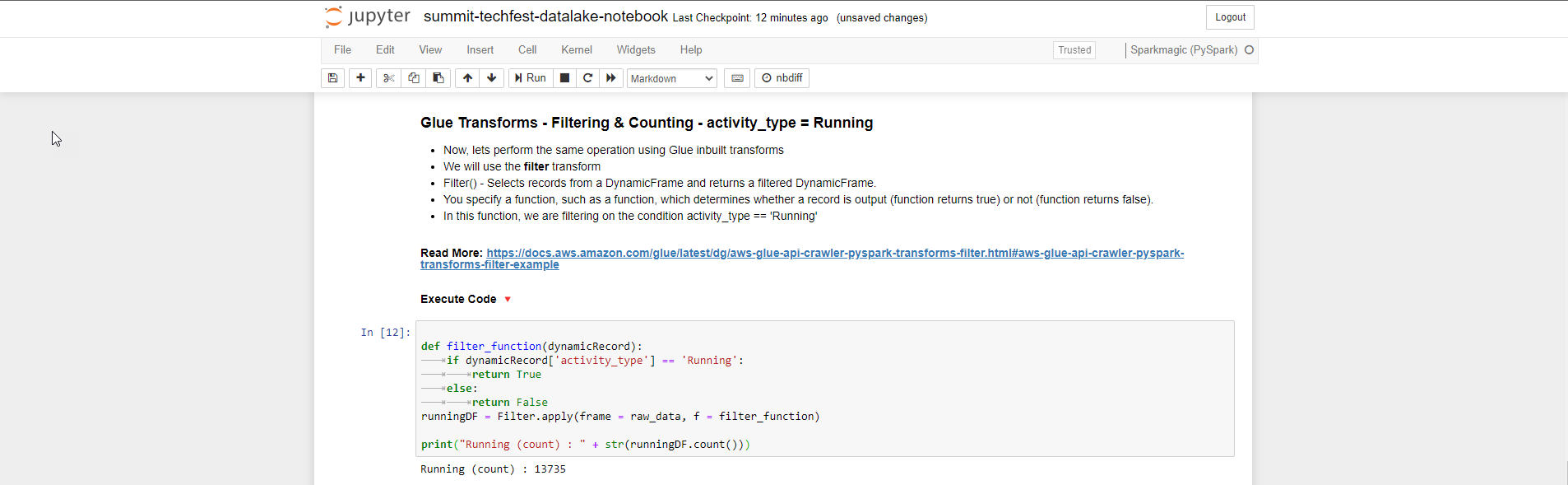1. Glue Transforms - Filtering & Counting - activity_type = Working using python Lambda Expressions
``````workingDF = Filter.apply(frame = raw_data, f = lambda x:x['activity_type']=='Working')

print("Working (count) : " + str(workingDF.count()))
``````1. Glue Transforms - Joining two dataframes, we join the dataframe to column track_id using apply() function and pass in frame1, frame2, key1, key2.
• You can refer to the document Read More
``````joined_data = Join.apply(raw_data,reference_data, 'track_id', 'track_id')
``````
• After joining, we will review the joined schema using printSchema() function
``````joined_data.printSchema()
``````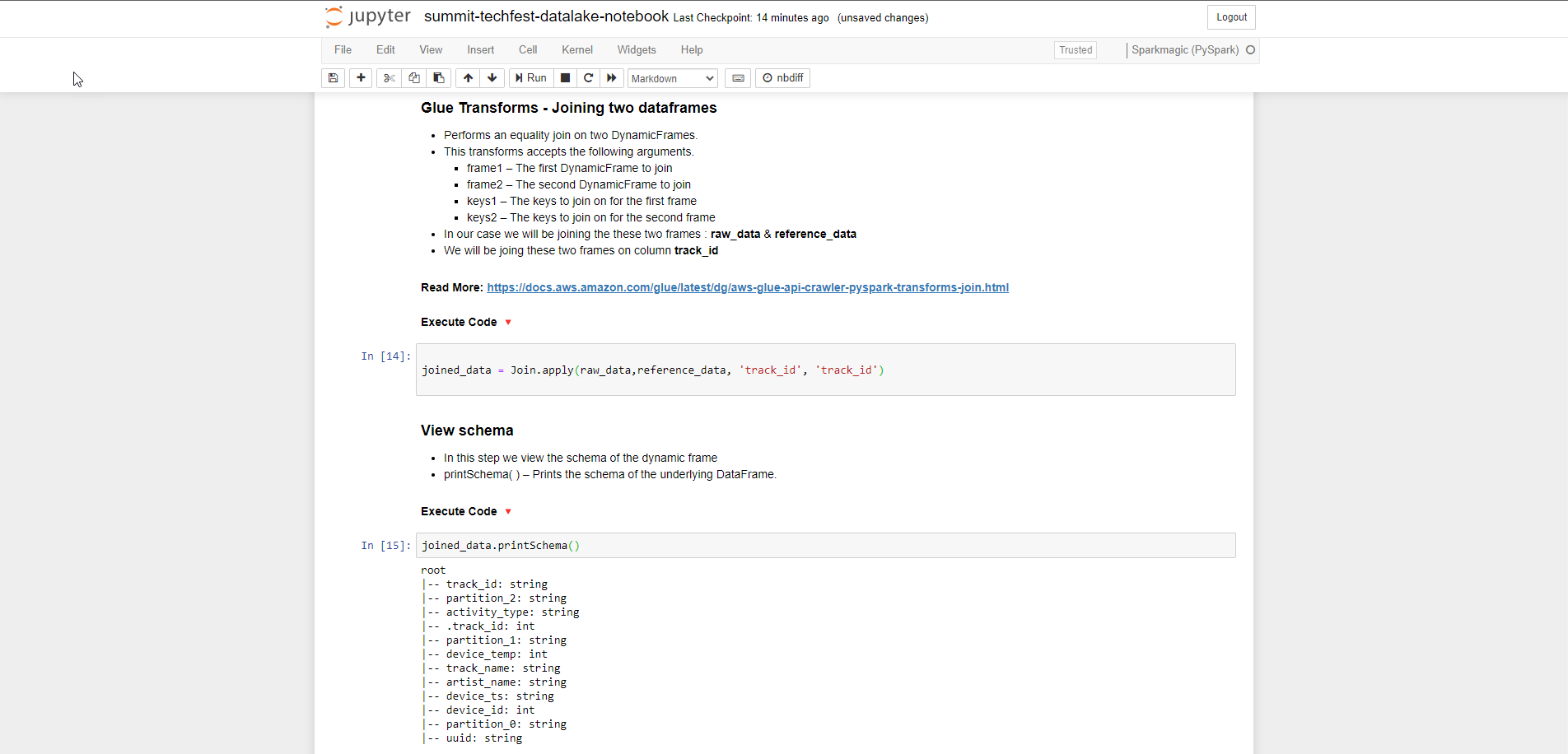1. We perform data cleaning
``````joined_data_clean = DropFields.apply(frame = joined_data, paths = ['partition_0','partition_1','partition_2','partition_3'])
``````1. Perform schema view after DropFields transform, switch to DataFrame and show data (show first 5 lines)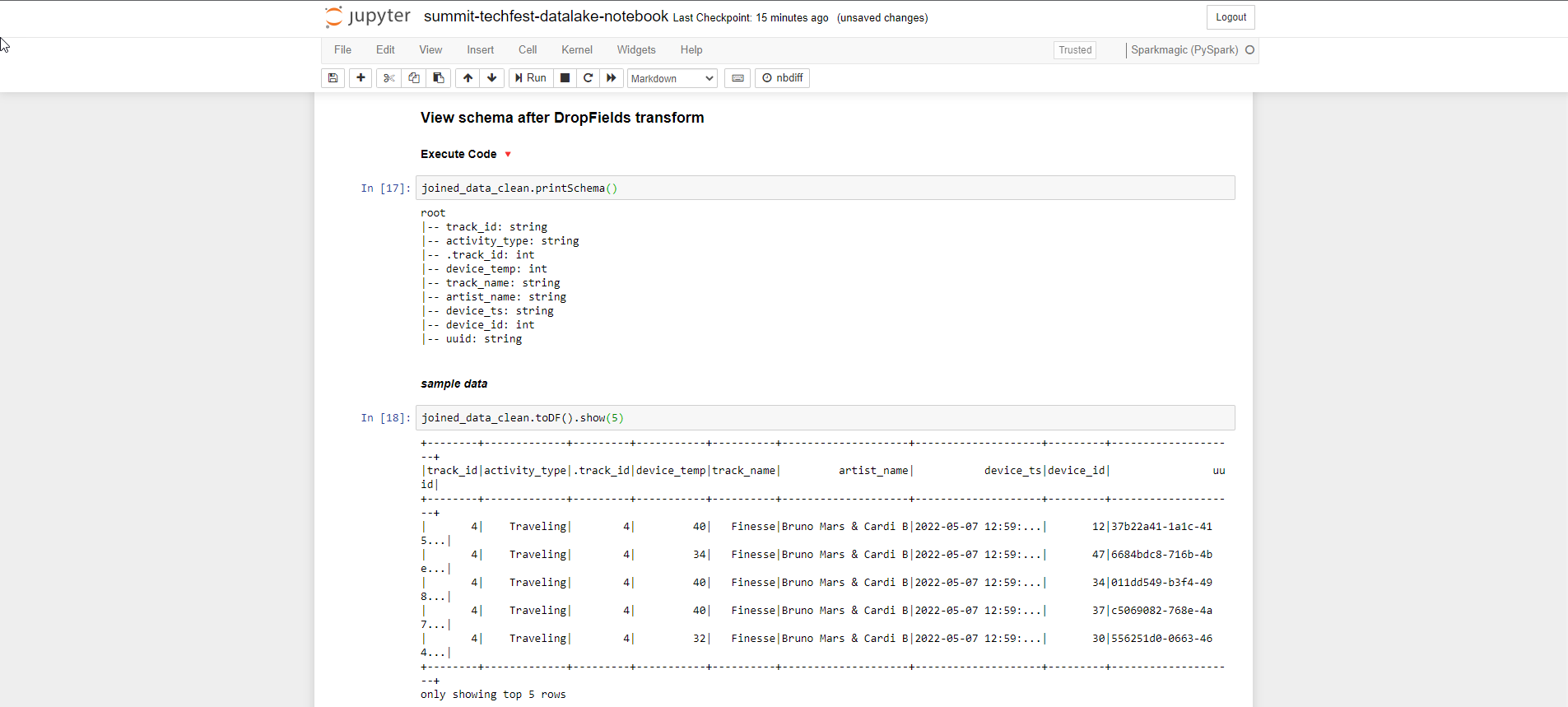1. The final step of transform is to write the data to S3 which stores it as parqet. You replace the path s3 bucket data instead of s3://yourname-datalake-demo-bucket/data/processed-data/
``````try:
datasink = glueContext.write_dynamic_frame.from_options(
frame = joined_data_clean, connection_type = "s3",
connection_options = {"path": "s3://yourname-datalake-demo-bucket/data/processed-data/"},
format = "parquet")
print('Transformed data written to S3')
except Exception as ex:
print('Something went wrong')
print(ex)
``````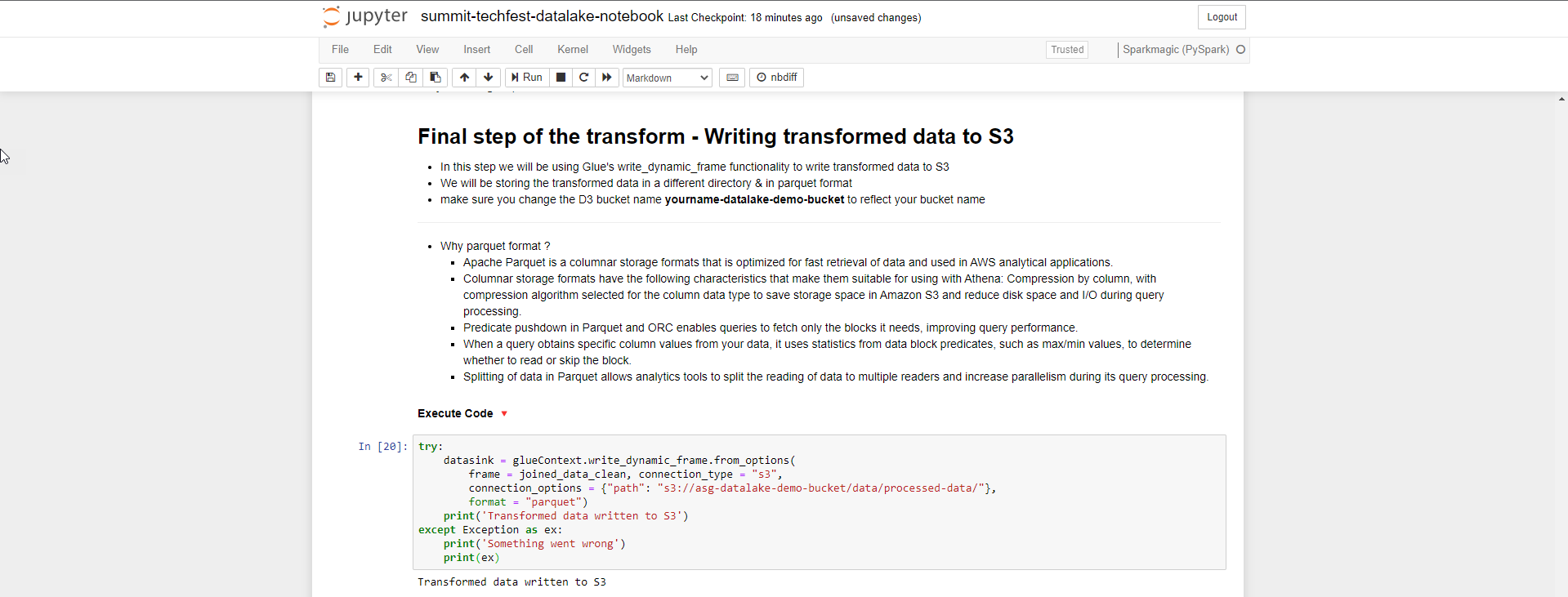1. Boto is the AWS SDK for Python. We use boto3 to run and automate AWS Glue. You change the Region name depending on the region you choose.
``````glueclient = boto3.client('glue',region_name='us-east-1')

response = glueclient.start_crawler(Name='summitcrawler')

print('---')

crawler_state = ''
while (crawler_state != 'STOPPING'):
response = glueclient.get_crawler(Name='summitcrawler')
crawler_state = str(response['Crawler']['State'])
time.sleep(1)

print('Crawler : Stopped')
print('---')
time.sleep(3)
``````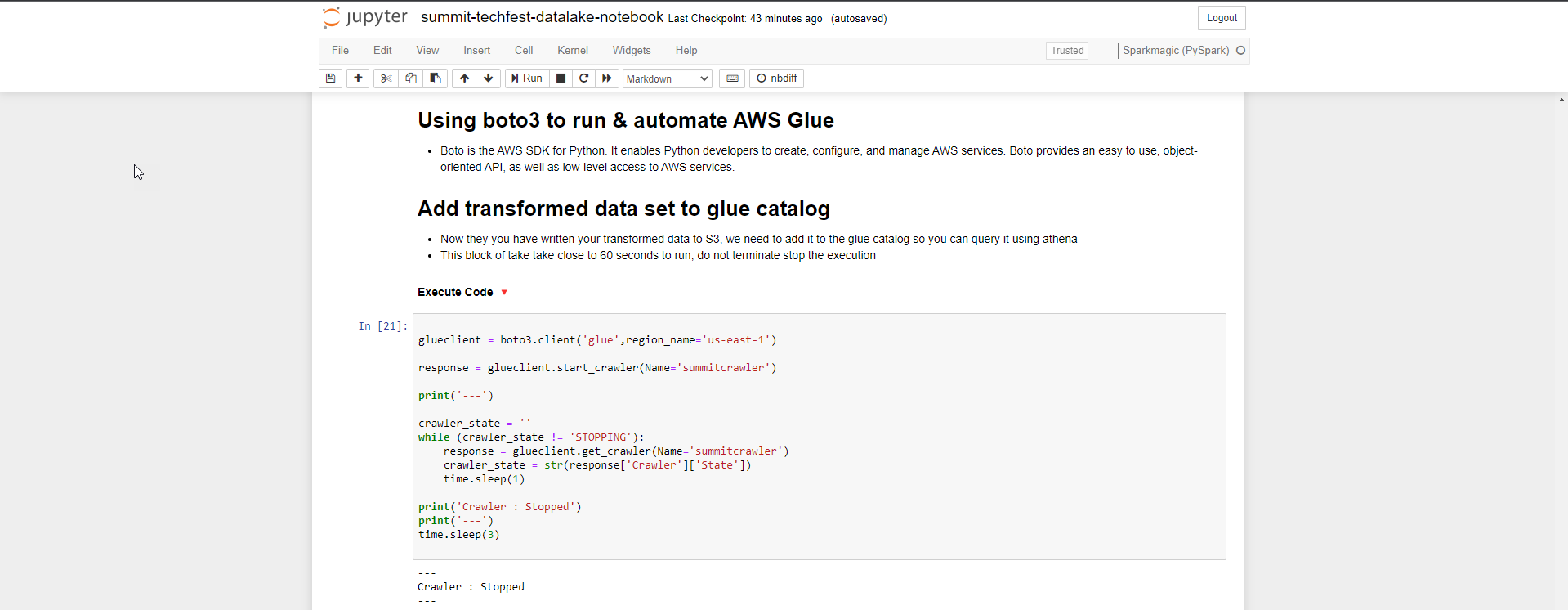1. After the steps, we have an overview of the list of tables in the summitdb database
``````print('** Summitdb has following tables**')
response = glueclient.get_tables(
DatabaseName='summitdb',
)

for table in response['TableList']:
print(table['Name'])
``````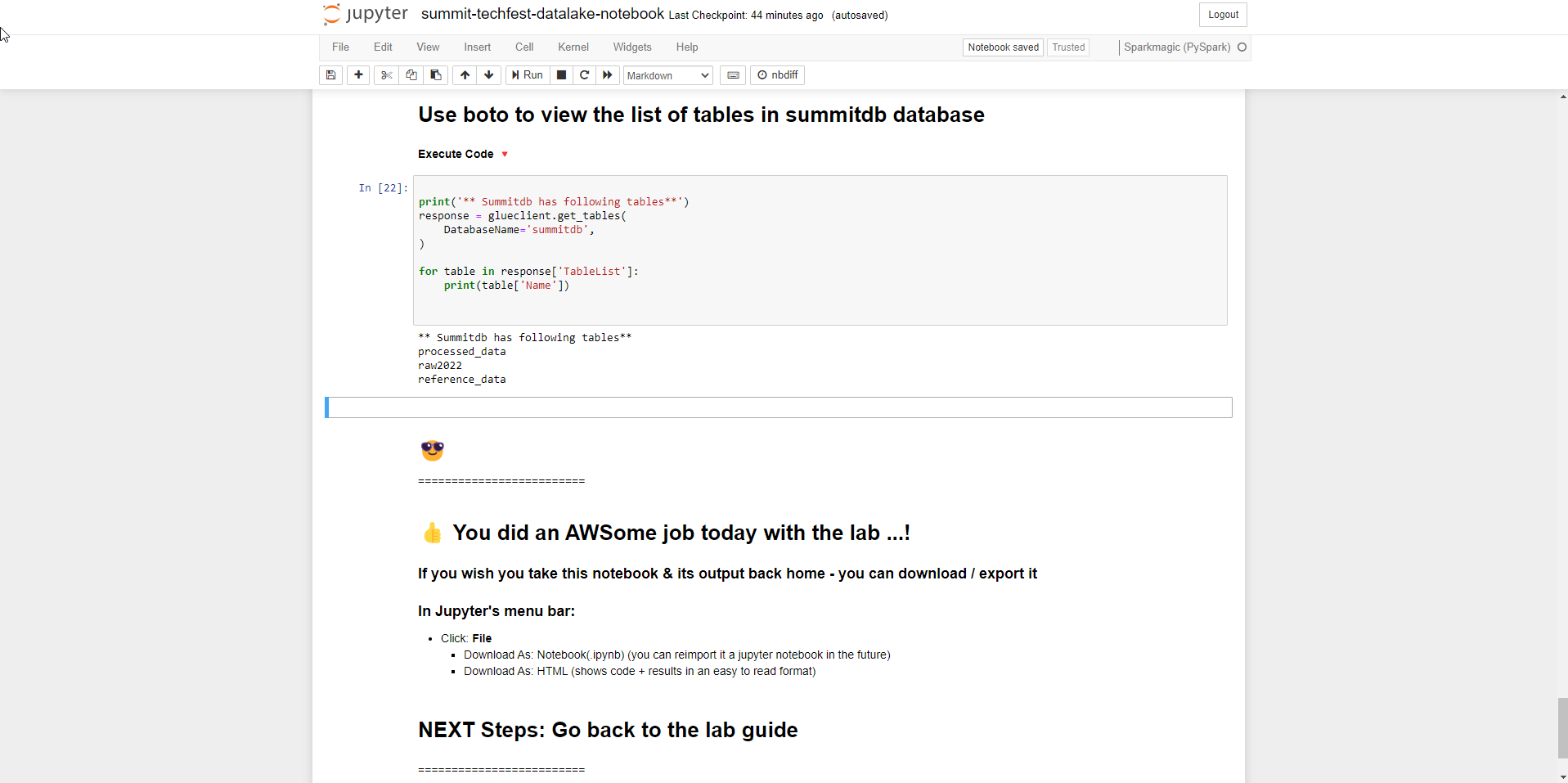1. Check if the data has been written to S3?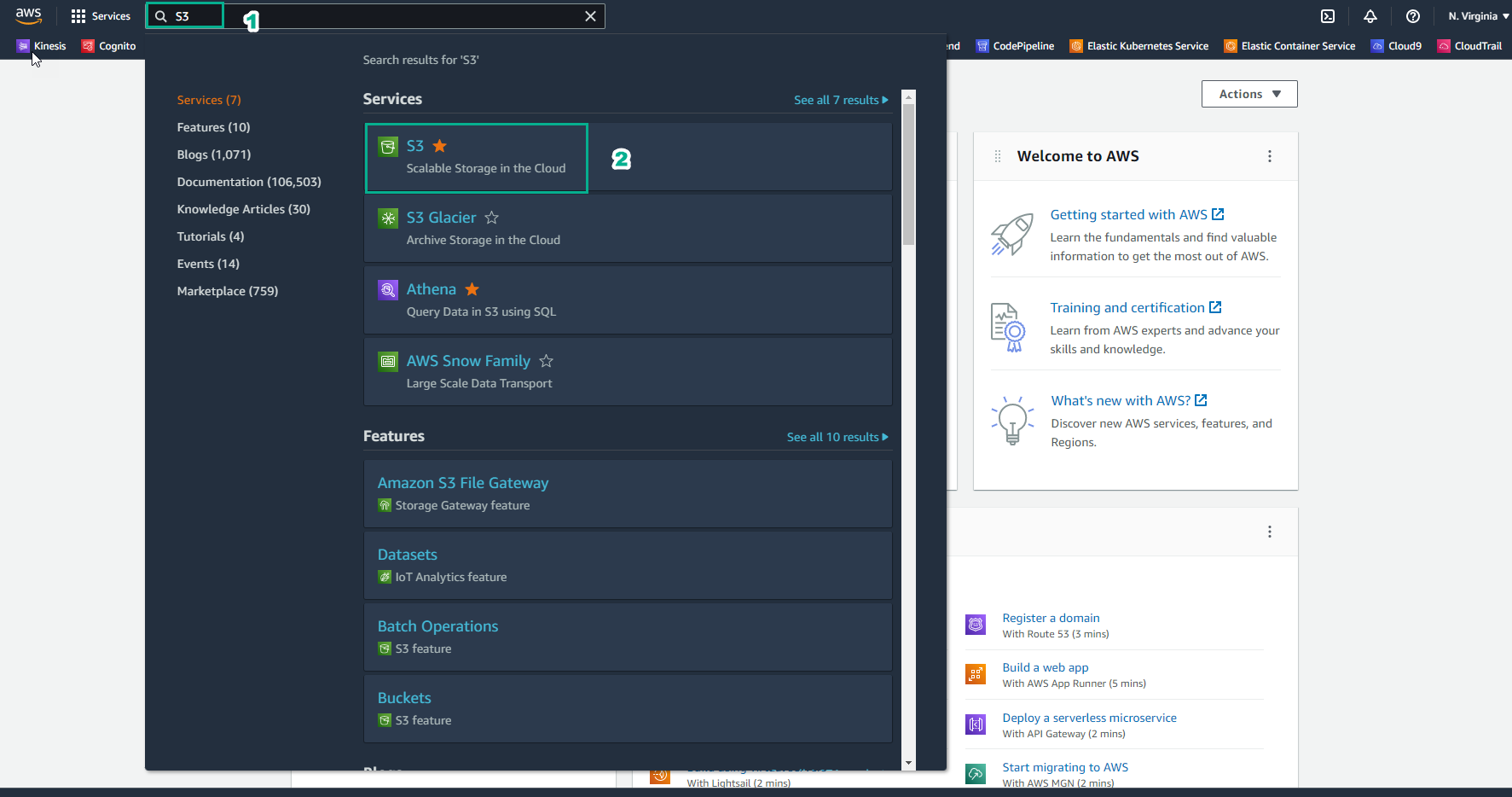1. In the S3 interface
• Select Buckets
• Select asg-datalake-demo-bucket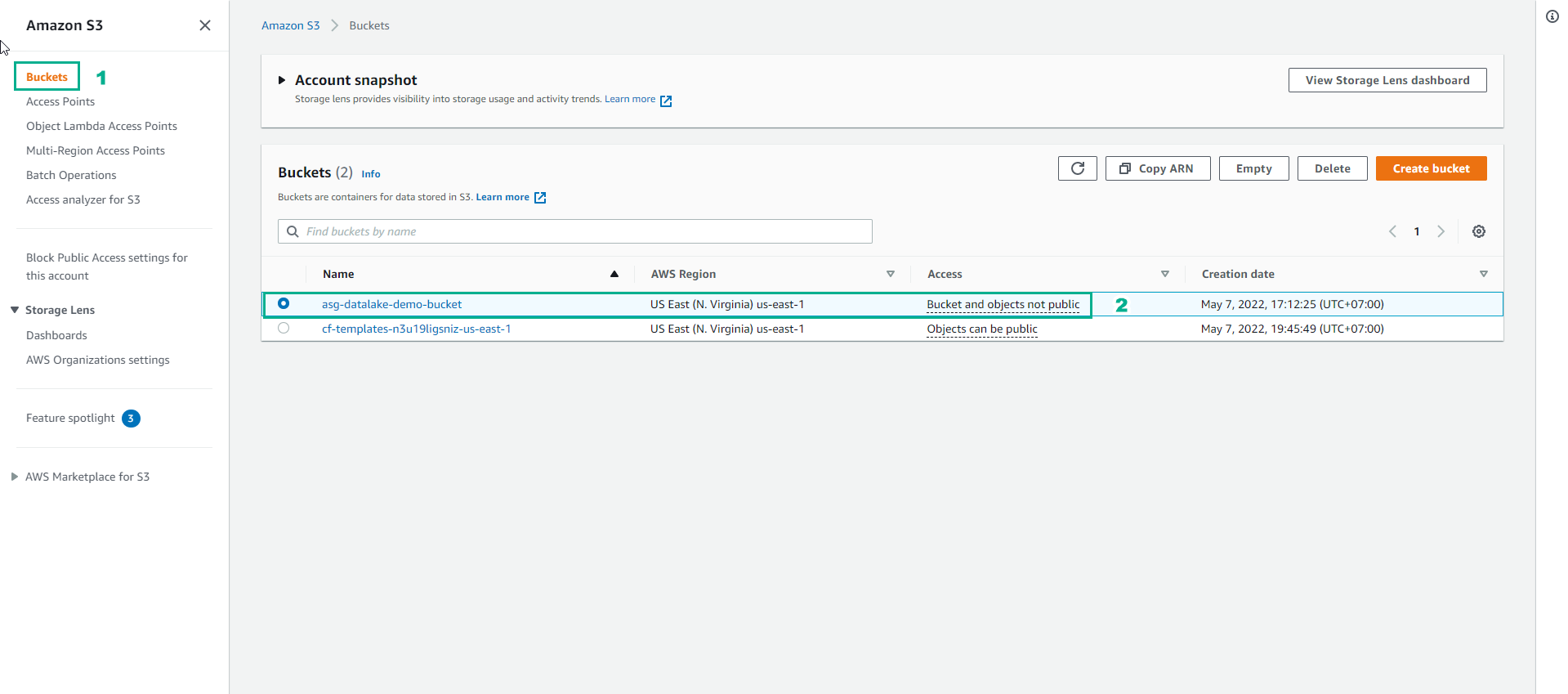1. Go to processed-data folder to see the recorded transform data